# Criteria for Similarity of Triangles

In Mathematics, a triangle is a closed two-dimensional figure or polygon with the least number of sides. A triangle has three sides and three angles. The most important property of a triangle is the sum of the interior angles of a triangle is equal to 180°. In this article, let us discuss the important criteria for the similarity of triangles with their theorem and proof and many solved examples.

## Conditions for Similarity of Two Triangles

Two triangles are said to be similar triangles,

• If their corresponding angles are equal.
• If their corresponding sides are in the same proportion/ratio.

Consider two triangles ABC and DEF.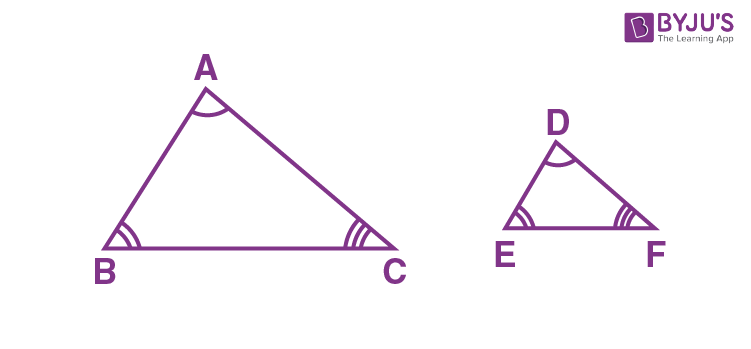The two triangles are said to be similar triangles, if

1. ∠A = ∠D, ∠B = ∠E, ∠C = ∠F
2. AB/DE = BC/EF = CA/FD

In this scenario, we can say that the two triangles ABC and DEF are similar.

## Important Criteria for Similarity of Triangles

The four important criteria used in determining the similarity of triangles are

• AAA criterion (Angle-Angle-Angle criterion)
• AA criterion (Angle-Angle criterion)
• SSS criterion (Side-Side-Side criterion)
• SAS Criterion (Side-Angle-Side criterion)

Now, let us discuss all these criteria for the similarity of triangles in detail.

### AAA Similarity Criterion for Two Triangles

The Angle-Angle-Angle (AAA) criterion for the similarity of triangles states that “If in two triangles, corresponding angles are equal, then their corresponding sides are in the same ratio (or proportion) and hence the two triangles are similar”.

Proof:

Consider two triangles ABC and DEF, such that ∠A = ∠D, ∠B = ∠E and ∠C = ∠F as shown in the figure.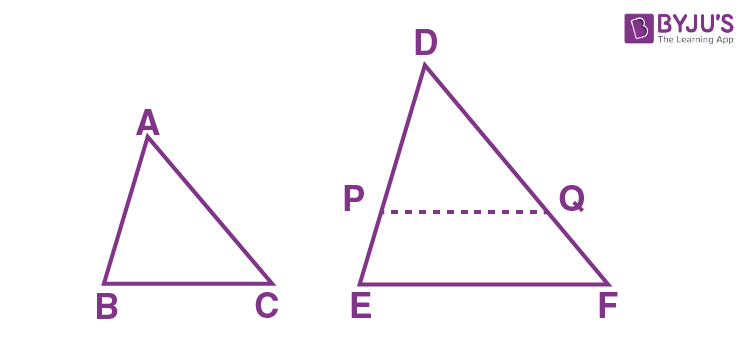Now, cut DP= AB and DQ= AC and join PQ. Hence, we can say that a triangle ABC is congruent to the triangle DPQ.

(i.e) ∆ ABC ≅ ∆ DPQ, which gives ∠B = ∠P = ∠E and also, the line PQ is parallel to EF.

Therefore, by using the basic proportionality theorem, we can write

DP/PE = DQ/QF.

(i.e) AB/DE = AC/DF. …(1)

Similarly,

AB/DE = BC/EF …(2)

From (1) and (2), we can write:

AB/DE = BC/EF = AC/DF.

Therefore, the two triangles ABC and DEF are similar.

### AA Similarity Criterion for Two Triangles

The Angle-Angle (AA) criterion for similarity of two triangles states that “If two angles of one triangle are respectively equal to two angles of another triangle, then the two triangles are similar”.

The AA criterion states that if two angles of a triangle are respectively equal to the two angles of another triangle, we can prove that the third angle will also be equal on both the triangles. This can be done with the help of the angle sum property of a triangle.

### SSS Similarity Criterion for Two Triangles

The Side-Side-Side (SSS) criterion for similarity of two triangles states that “If in two triangles, sides of one triangle are proportional to (i.e., in the same ratio of ) the sides of the other triangle, then their corresponding angles are equal and hence the two triangles are similar”.

Proof:

Consider the same figure as given above. It is observed that DP/PE = DQ/QF and also in the triangle DEF, the line PQ is parallel to the line EF.

So, ∠P = ∠E and ∠Q = ∠F.

Hence, we can write: DP/DE = DQ/DF= PQ/EF.

The above expression is written as

DP/DE = DQ/DF=BC/EF.

It means that PQ = BC.

Hence, the triangle ABC is congruent to the triangle DPQ.

(i.e) ∆ ABC ≅ ∆ DPQ.

Thus, by using the AAA criterion for similarity of the triangle, we can say that

∠A = ∠D, ∠B = ∠E and ∠C = ∠F.

### SAS Similarity Criterion for Two Triangles

The Side-Angle-Side (SAS) criterion for similarity of two triangles states that “If one angle of a triangle is equal to one angle of the other triangle and the sides including these angles are proportional, then the two triangles are similar”.

Proof:

This theorem can be proved by taking two triangles such as ABC and DEF (Refer to the same figure as given above).

By using the basic proportionality theorem, we get

AB/DE = AC/DF and ∠A = ∠D

In the triangle DEF, the line PQ is parallel to EF.

So, ∆ ABC ≅ ∆ DPQ.

Hence, we can say ∠A = ∠D, ∠B=∠P and ∠C= ∠Q, which means that the triangle ABC is similar to the triangle DEF.

(i.e) ∆ ABC ~ ∆ DEF.

### Examples

Now, let us use the criteria for the similarity of triangles to find the unknown angles and sides of a triangle.

Example 1:

Find ∠P in the following triangles.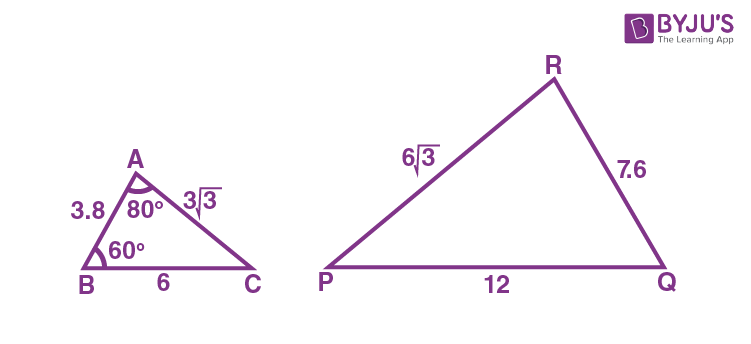Solution:

From the given triangles, ABC and PQR, we can get

AB/RQ = 3.8/7.6 = ½

BC/QP = 6/12 = ½

CA/PR = (3√3)/6√3 = ½

Therefore,

AB/RQ = BC/QP = CA/PR

Hence, by using the SSS similarity criterion for a triangle, we can write

∆ ABC ~ ∆ RQP (i.e) ∆ABC is similar to ∆RQP.

By using corresponding angles of similar triangles,

∠C = ∠P

∠C = 180°- ∠A – ∠B (Using the angle sum property of triangle).

∠C= 180° – 80° – 60°

∠C= 40°.

Since, ∠C= ∠P, the value of ∠p is 40°.

Example 2:

Show that the triangles POQ and SOR are similar triangles, given that PQ is parallel to RS as shown in the figure.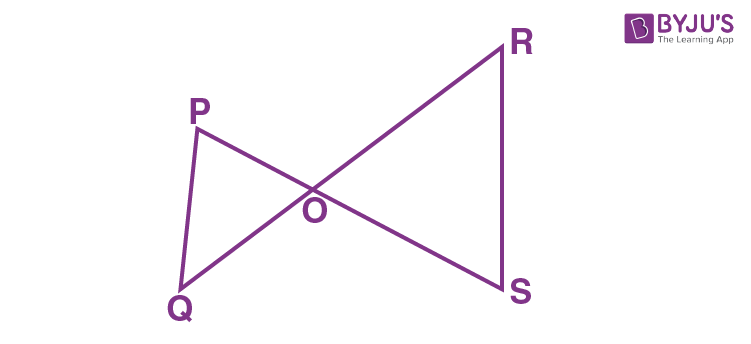Solution:

Given that PQ is parallel to RS. (i.e) PQ || RS.

By using alternate angles property, ∠P = ∠S and ∠Q = ∠R.

Also, by using the vertically opposite angles, ∠POQ = ∠SOR.

Hence, we can conclude that a triangle POQ is similar to the triangle SOR.

(i.e) ∆ POQ ~ ∆ SOR (Using AAA similarity criterion for triangles)

Hence, proved.

## Video Lesson on BPT and Similar Triangles### Practice Problems

1. From the given figure, if ∆ ABE ≅ ∆ ACD, prove that ∆ ADE ~ ∆ ABC.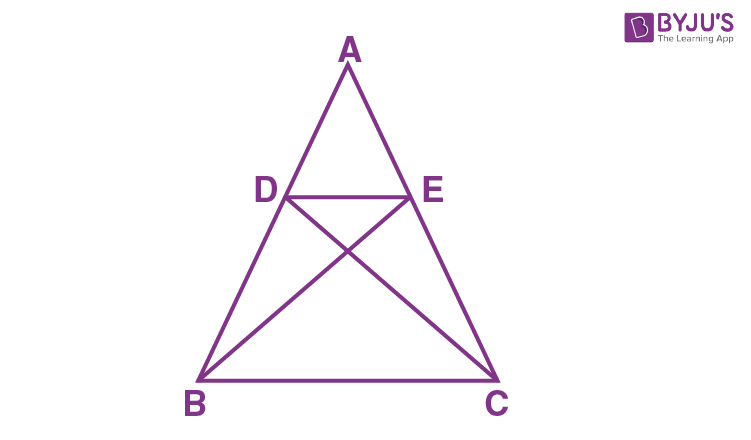2. In the given figure, QR/QS = QT/PR and ∠1= ∠2. Show that ∆ PQS ~ ∆ TQR.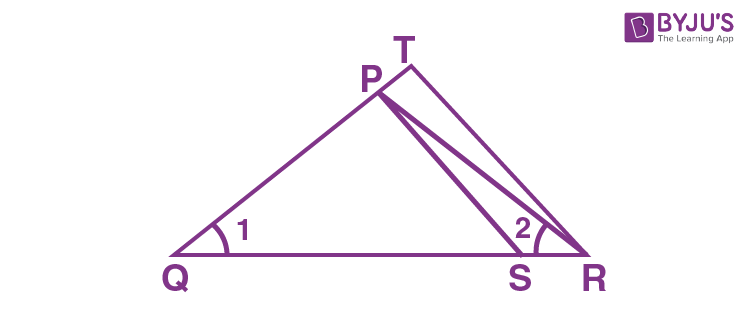3. Determine the height of a tower, if a vertical pole of length 6 m casts a shadow 4 m long on the ground and at the same time a tower casts a shadow 28 m long.

Keep visiting BYJU’S – The Learning App and download the app to learn all Maths-related concepts by exploring more videos.